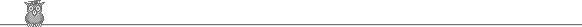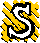### 10th Putnam 1950A1.  a and b are positive reals and a > b. Let C be the plane curve r = a - b cos θ. For what values of b/a is C convex?A2.  Does the series ∑2∞ 1/ln n! converge? Does the series 1/3 + 1/(3 31/2) + 1/(3 31/2 31/3) + ... + 1/(3 31/2 31/3 ... 31/n) + ... converge?A3.  The sequence an is defined by a0 = α, a1 = β, an+1 = an + (an-1 - an)/(2n). Find lim an.A4.  Do either (1) or (2) (1)   P is a prism with triangular base. A is a vertex. The total area of the three faces containing A is 3k. Show that if the volume of P is maximized, then each of the three faces has area k and the two lateral faces are perpendicular to each other. (2)   Let f(x) = x + x3/(1·3) + x5/(1·3·5) + x7/(1·3·5·7) + ... , and g(x) = 1 + x2/2 + x4/(2·4) + x6/(2·4·6) + ... . Show that ∫0x exp( - t2/2) dt = f(x)/g(x).A5.  Let N be the set of natural numbers {1, 2, 3, ... }. Let Z be the integers. Define d : N → Z by d(1) = 0, d(p) = 1 for p prime, and d(mn) = m d(n) + n d(m) for any integers m, n. Determine d(n) in terms of the prime factors of n. Find all n such that d(n) = n. Define d1(m) = d(m) and dn+1(m) = d(dn(m)). Find limn→∞ dn(63).A6.  Let f(x) = ∑0∞ anxn and suppose that each an = 0 or 1. Do either (1) or (2): (1)   Show that if f(1/2) is rational, then f(x) has the form p(x)/q(x) for some integer polynomials p(x) and q(x). (2)   Show that if f(1/2) is not rational, then f(x) does not have the form p(x)/q(x) for any integer polynomials p(x) and q(x).B1.  Given n, not necessarily distinct, points P1, P2, ... , Pn on a line. Find the point P on the line to minimize ∑ |PPi|.B2.  An ellipse with semi-axes a and b has perimeter length p(a, b). For b/a near 1, is π(a + b) or 2π√(ab) the better approximation to p(a, b)?B3.  Leap years have 366 days; other years have 365 days. Year n > 0 is a leap year iff (1) 4 divides n, but 100 does not divide n, or (2) 400 divides n. n is chosen at random from the natural numbers. Show that the probability that December 25 in year n is a Wednesday is not 1/7.B4.  A long, light cylinder has elliptical cross-section with semi-axes a > b. It lies on the ground with its main axis horizontal and the major axes horizontal. A thin heavy wire of the same length as the cylinder is attached to the line along the top of the cylinder. [We could take the cylinder to be the surface |x| ≤ L, y2/a2 + z2/b2 = 1. Contact with the ground is along |x| ≤ L, y = 0, z = -b. The wire is along |x| ≤ L, y = 0, z = b.] For what values of b/a is the cylinder in stable equilibrium?B5.  Do either (1) or (2): (1)   Show that if ∑(an + 2an+1) converges, then so does ∑ an. (2)   Let S be the surface 2xy = z2. The surface S and the variable plane P enclose a cone with volume πa3/3, where a is a positive real constant. Find the equation of the envelope of P. What is the envelope in the case of a general cone?B6.  (1)   The convex polygon C' lies inside the polygon C. Is it true that the perimeter of C' is no longer than the perimeter of C? (2)   C is the convex polygon with shortest perimeter enclosing the polygon C'. Is it true that the perimeter of C is no longer than the perimeter of C' ? (3)   The closed convex surface S' lies inside the closed surface S. Is it true that area S' ≤ area S? (4)   S is the smallest convex surface containing the closed surface S'. Is it true that area S ≤ area S'?To avoid possible copyright problems, I have changed the wording, but not the substance, of all the problems. The original text and solutions are available in: A M Gleason, R E Greenwood & L M Kelly, The William Lowell Putnam Mathematical Competition, Problems and Solutions, 1938-1964, MAA 1980. Out of print, but available in some university libraries.

Putnam home